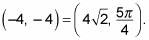##### Linear Algebra For Dummies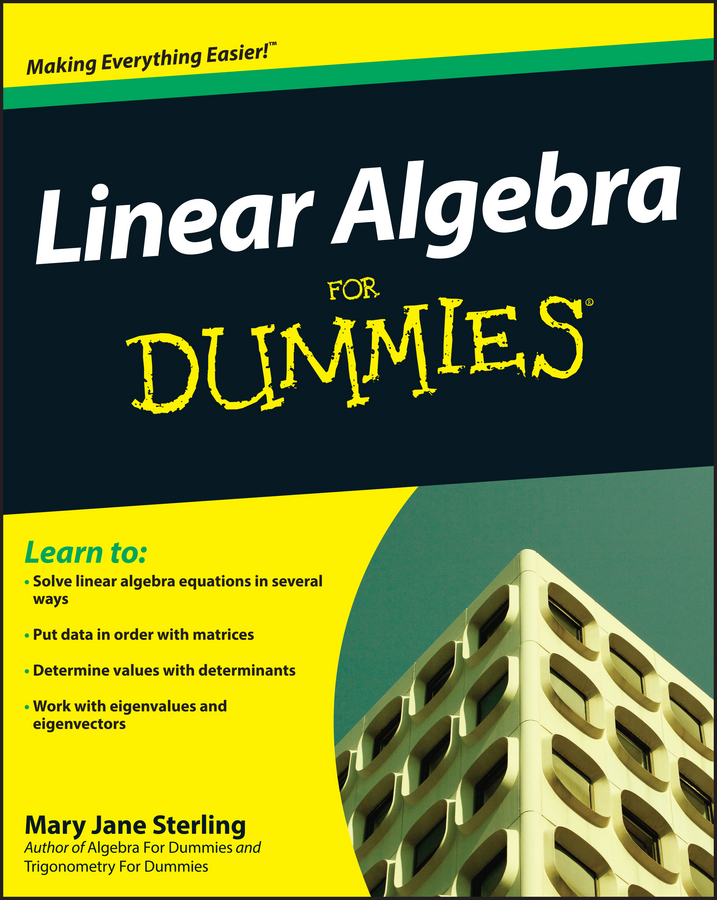You can use both polar coordinates and Cartesian (x, y) coordinates (also known as rectangular coordinates) at any time to describe the same location on the coordinate plane. Sometimes you'll have an easier time using one form, and for this reason it's important to know how to change between the two. Cartesian coordinates are much better suited for graphs of straight lines or simple curves. Polar coordinates can yield you a variety of pretty, very complex graphs that you can't plot with Cartesian coordinates.

When changing to and from polar coordinates, your work is often easier if you have all your angle measures in radians. You can make the change by using the conversion factor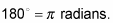You may choose, however, to leave your angle measures in degrees, which is fine as long as your calculator is in the right mode.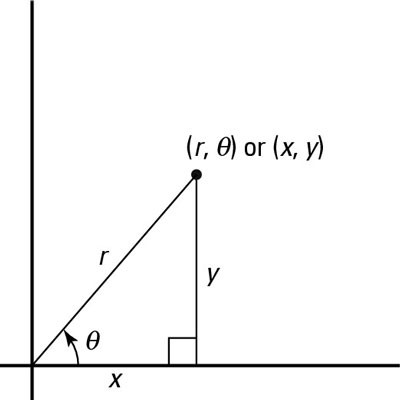A polar and (x, y) coordinate mapped in the same plane.

Examine the point in this figure, which illustrates a point mapped out in both (x, y) and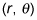coordinates, allowing you to see the relationship between them.

What exactly is the geometric relationship between r,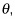x, and y? Look at how they're labeled on the graph — all parts of the same triangle!

Using right-triangle trigonometry, you know the following facts: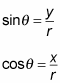These equations simplify into two very important expressions for x and y in terms of r and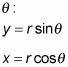Furthermore, you can use the Pythagorean theorem in the right triangle to find the radius of the triangle if given x and y:

x2 + y2 = r2

One final equation allows you to find the angle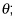it derives from the tangent of the angle: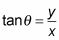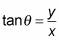So if you solve this equation foryou get the following expression: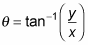With respect to the final equation, keep in mind that your calculator always returns a value of inverse tangent that puts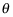in the first or fourth quadrant. You need to look at your x- and y-coordinates and decide whether that placement is actually correct for the problem at hand. Your calculator doesn't look for tangent possibilities in the second and third quadrants, but that doesn't mean you don't have to!

Together, the four equations for r,x, and y allow you to change (x, y) coordinates into polarcoordinates and back again anytime. For example, to change the polar coordinate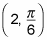to a rectangular coordinate, follow these steps:

1. Find the x value.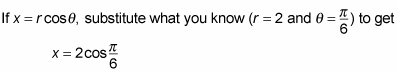Use the unit circle to get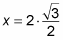which means that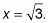2. Find the y value.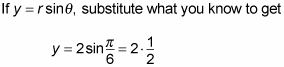which means that y = 1.

3. Express the values from Steps 1 and 2 as a coordinate point.

You find that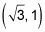is the answer as a point.

Time for an example in reverse. Given the point (–4, –4), find the equivalent polar coordinate:

1. Plot the (x, y) point first.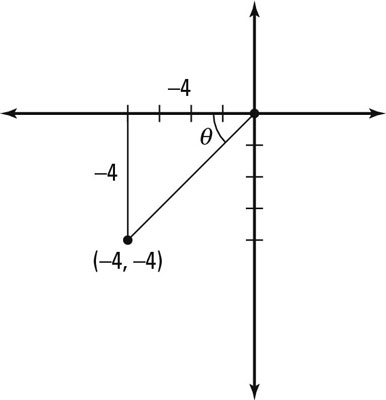An (x, y) coordinate changed to a polar coordinate.

This figure shows the location of the point in quadrant III.

2. Find the r value.

For this step, you use the Pythagorean theorem for polar coordinates: x2 + y2 = r2. Plug in what you know (x = –4 and y = –4) to get (–4)2 + (–4)2 = r2, or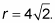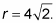3. Find the value of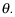Use the tangent ratio for polar coordinates: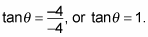The reference angle for this value is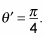You know from the figure that the point is in the third quadrant, so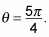4. Express the values of Steps 2 and 3 as a polar coordinate.

You can say that